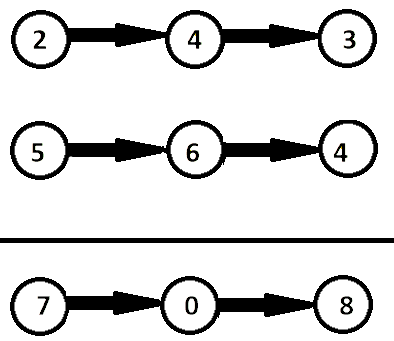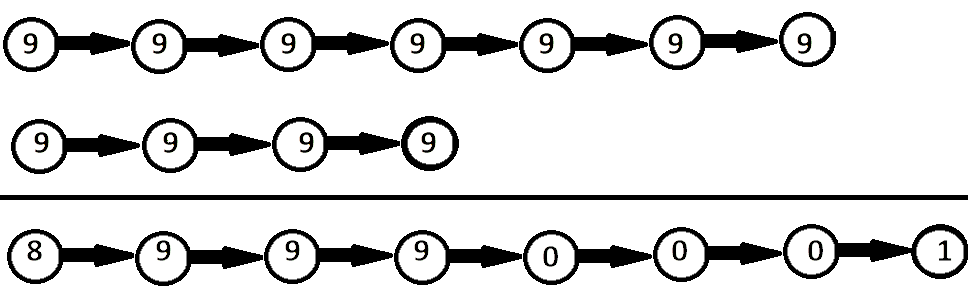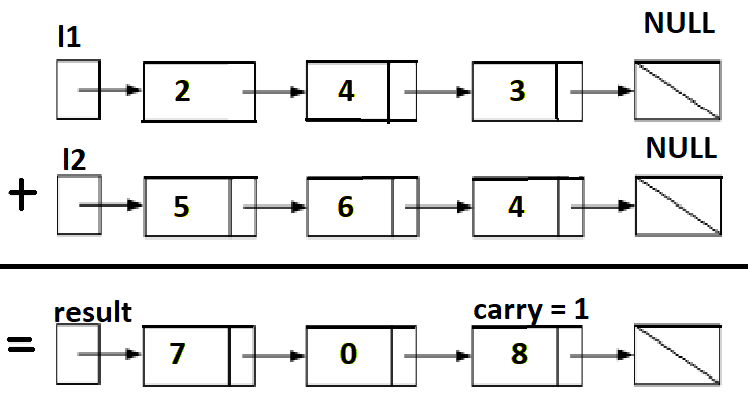# Add two numbers represented as Linked Lists

Problem Statement: Given the heads of two non-empty linked lists representing two non-negative integers. The digits are stored in reverse order, and each of their nodes contains a single digit. Add the two numbers and return the sum as a linked list.

Examples:

```Input Format:

num1  = 243, num2 = 564

l1 = [2,4,3]
l2 = [5,6,4]

Result: sum = 807; L = [7,0,8]

Explanation: Since the digits are stored in reverse order, reverse the numbers first to get the or                                                original number and then add them as → 342 + 465 = 807. Refer to the image below.``````Input Format:

l1 = [9,9,9,9,9,9,9], l2 = [9,9,9,9]

Result: [8,9,9,9,0,0,0,1]

Explanation: Since the digits are stored in reverse order, reverse the numbers first to get the     original number and then add them as → 9999999 + 9999 = 8999001. Refer to the image below.```### Solution

Disclaimer: Don’t jump directly to the solution, try it out yourself first.

Solution 1: Elementary Math

Intuition: Keep track of the carry using a variable and simulate digits-by-digits sum starting from the head of the list, which contains the least significant digit.

ApproachVisualization of the addition of two numbers:

342 + 465 = 807

342+465=807.

Each node contains a single digit and the digits are stored in reverse order.

Just like how you would sum two numbers on a piece of paper, we begin by summing the least significant digits, which is the head of l1 and l2. Since each digit is in the range of 0…9, summing two digits may “overflow”. For example

5 + 7 = 12. In this case, we set the current digit to 2 and bring over the carry=1 to the next iteration.

carry must be either 0 or 1 because the largest possible sum of two digits (including the carry) is 9 + 9 + 1 = 19.

Psuedocode:

• Create a dummy node which is the head of new linked list.
• Create a node temp, initialise it with dummy.
• Initialize carry to 0.
• Loop through lists l1 and l2 until you reach both ends, and until carry is present.
• Set sum=l1.val+ l2.val + carry.
• Update carry=sum/10.
• Create a new node with the digit value of (sum%10) and set it to temp node’s next, then advance temp node to next.
• Advance both l1 and l2.
• Return dummy’s next node.

Note that we use a dummy head to simplify the code. Without a dummy head, you would have to write extra conditional statements to initialize the head’s value.

Take extra caution in the following cases:

Code:

## C++ Code

``````/**
* Definition for singly-linked list.
* struct ListNode {
*     int val;
*     ListNode *next;
*     ListNode() : val(0), next(nullptr) {}
*     ListNode(int x) : val(x), next(nullptr) {}
*     ListNode(int x, ListNode *next) : val(x), next(next) {}
* };
*/
class Solution {
public:
ListNode* addTwoNumbers(ListNode* l1, ListNode* l2) {
ListNode *dummy = new ListNode();
ListNode *temp = dummy;
int carry = 0;
while( (l1 != NULL || l2 != NULL) || carry) {
int sum = 0;
if(l1 != NULL) {
sum += l1->val;
l1 = l1 -> next;
}

if(l2 != NULL) {
sum += l2 -> val;
l2 = l2 -> next;
}

sum += carry;
carry = sum / 10;
ListNode *node = new ListNode(sum % 10);
temp -> next = node;
temp = temp -> next;
}
return dummy -> next;
}
};``````

## Java Code

``````/**
* Definition for singly-linked list.
* public class ListNode {
*     int val;
*     ListNode next;
*     ListNode() {}
*     ListNode(int val) { this.val = val; }
*     ListNode(int val, ListNode next) { this.val = val; this.next = next; }
* }
*/
class Solution {
public ListNode addTwoNumbers(ListNode l1, ListNode l2) {
ListNode dummy = new ListNode();
ListNode temp = dummy;
int carry = 0;
while( l1 != null || l2 != null || carry == 1) {
int sum = 0;
if(l1 != null) {
sum += l1.val;
l1 = l1.next;
}

if(l2 != null) {
sum += l2.val;
l2 = l2.next;
}

sum += carry;
carry = sum / 10;
ListNode node = new ListNode(sum % 10);
temp.next = node;
temp = temp.next;
}
return dummy.next;
}
}``````

Time Complexity: O(max(m,n)). Assume that m and n represent the length of l1 and l2 respectively, the algorithm above iterates at most max(m,n) times.

Space Complexity: O(max(m,n)). The length of the new list is at most max(m,n)+1.

Special thanks to Anshu Patidar for contributing to this article on takeUforward. If you also wish to share your knowledge with the takeUforward fam, please check out this article.